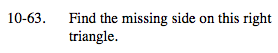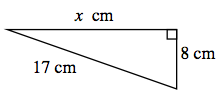### Home > MC2 > Chapter 10 > Lesson 10.1.5 > Problem10-63

10-63.Since this is a right trianlgle, we can use the Pythagorean Theorem to find the missing side length.

(leg 1)2 + (leg 2)2 = (hypotenuse)2
We know one of the leg lengths and the hypotenuse. Substitute the numbers into the equation.

x2 + (8)2 = 172
Now solve for x.